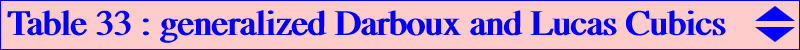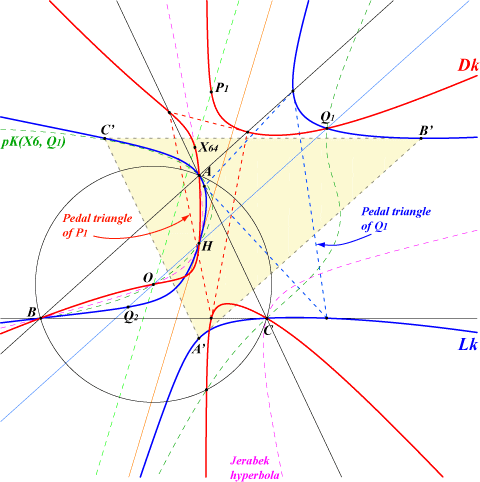This page has its roots in several Hyacinthos messages (#14861 & sq.) by Quang Tuan Bui and also Fred Lang. Let k be a real number and h the homothety with center H, ratio k. The image of the reference triangle ABC under h is A'B'C'. The pedal triangle of a point X and A'B'C' are perspective (at Y) if and only if X lies on a cubic denoted by Dk and, in this case, the perspector Y lies on another cubic denoted by Lk. When k = 1, the triangles ABC and A'B'C' coincide and the corresponding cubics D1, L1 are the Darboux cubic K004 and the Lucas cubic K007 respectively (see property 1 in each page). For this reason, Dk and Lk are called the generalized Darboux and Lucas cubics. Equations Let F0(x,y,z) = ∑ a^2(b^2 - c^2)SA yz, where F0 = 0 is the Jerabek hyperbola, F1(x,y,z) = ∑ [SA(SB+SC) - SB SC] x(c^2y - b^2z^2), where F1 = 0 is the Darboux cubic, F2(x,y,z) = ∏ (SB y - SC z), where F2 = 0 is the union of the altitudes of ABC, F3(x,y,z) = ∑ x^2(SB y - SC z), where F3 = 0 is the Lucas cubic. The equation of Dk is (1) : 2 F1 - (k - 1)(x + y + z) F0 = 0, and the equation of Lk is (2) : 4k (k - 1)(x + y + z) F0 + (k - 1) F2 + 16 ∆^2 k F3 = 0 ( ∆ is the area of ABC). Properties of Dk (1) clearly shows that the cubics Dk form a pencil generated by the Darboux cubic itself (obtained for k = 1) and the decomposed cubic which is the union of the Jerabek hyperbola and the line at infinity (the limit case k = ∞). It follows that : — Dk is a circumcubic. The tangents at A, B, C concur if and only if k = 0 (the corresponding cubic D0 is K376), k = 1 (Darboux cubic), k = -1. — Dk has three real asymptotes parallel to the altitudes of ABC. These concur if and only if k = 1 (Darboux cubic). Dk contains : — O, H, X(64) isogonal conjugate of the de Longchamps point X(20). — P1, the image of X(64) under the homothety h(O, -k). P1 is the tangential of H in Dk. — the vertices of the pedal triangle of P1. — Q1, the image of X(20) under the homothety h hence the point X(20) in A'B'C'. Q1 is also the perspector of A'B'C' and the pedal triangle of P1. — the common points of the circumcircle and the cubic pK(K, Q1), a member of the Euler pencil of cubics. The polar conic of any point on the Euler line is a rectangular hyperbola and there is no other point of the plane having this property. Properties of Lk (2) shows that the cubics Lk form a family (not a pencil) which contains the Lucas cubic (obtained for k = 1), the decomposed cubic which is the union of the altitudes of ABC (the limit case k = 0) and the decomposed cubic which is the union of the Jerabek hyperbola and the line at infinity (the limit case k = ∞). See below for other special cubics Lk. Lk contains : — A, B, C. The tangents at A, B, C concur if and only if k = 0, k = 1, k = 1/2 and three other values giving three decomposed cubics. — H where the tangent is the line H-X(51) for any k. — A', B', C'. The tangents at A', B', C' concur if and only if k = 0, k = 1 and two other rather complicated values. — Q1 and the vertices of its pedal triangle. — Q2, perspector of the medial triangle (pedal triangle of O) and A'B'C'. Q2 is the third point on the Euler line. It is given by OQ2 = 1 / (2k+1) OH (vectors). — Q3, perspector of the pedal triangle of X(64) and A'B'C'. — Q4, perspector of the pedal triangle of Q1 and A'B'C'. — three other points on the circumcircle which always lies on an isogonal pK with pivot on a hyperbola passing through X(5) (when k = 1/2, see below), X(30), X(2979) (when k = 1). The polar conic of any point on the Euler line is again a rectangular hyperbola and there is no other point of the plane having this property except when k = 1/2. This latter case gives the only equilateral cubic of the family which is the Musselman (first) cubic K026. If k ≠ 1/2, the rectangular hyperbolas which are the polar conics of H are all centered on a same rectangular hyperbola passing through X(4), X(74), X(107), X(125), X(133), X1204), X(2777). Special cubics Lk and related cubics Dk This family of cubics contains : — two K0 (without term in x y z) when k = 0 (union of the altitudes of ABC) and k = 1 (Lucas cubic K007). Thus, K007 is the only pK of the family. — one equilateral cubic when k = 1/2. This is K026. The corresponding cubic Dk is K405. — one circular cubic when k = -1/2. This is K427. — three K+ (with concurring asymptotes) when k = 0 (union of the altitudes), k = 1/2 (giving K026 again) and k = - 1 (giving the Quang Tuan Bui cubic K425, called C2 in the Hyacinthos message cited above, the corresponding cubic Dk is not very interesting here). In fact, these three cubics K+ are all central cubics K++.